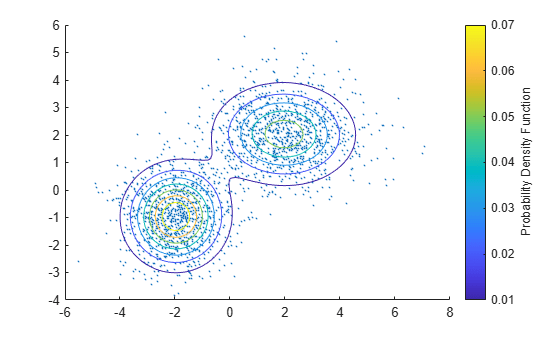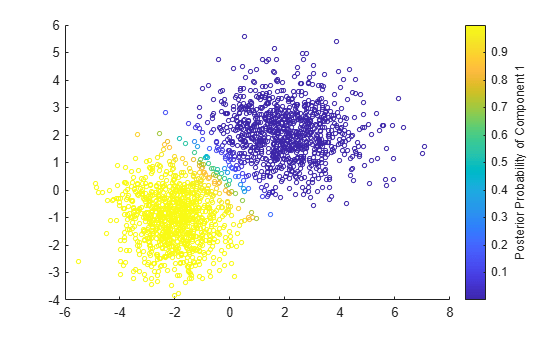# posterior

Posterior probability of Gaussian mixture component

## Syntax

``P = posterior(gm,X)``
``[P,nlogL] = posterior(gm,X)``

## Description

example

````P = posterior(gm,X)` returns the posterior probability of each Gaussian mixture component in `gm` given each observation in `X`.```
````[P,nlogL] = posterior(gm,X)` also returns the negative loglikelihood of the Gaussian mixture model `gm` given the data `X`.```

## Examples

collapse all

Generate random variates that follow a mixture of two bivariate Gaussian distributions by using the `mvnrnd` function. Fit a Gaussian mixture model (GMM) to the generated data by using the `fitgmdist` function, and then compute the posterior probabilities of the mixture components.

Define the distribution parameters (means and covariances) of two bivariate Gaussian mixture components.

```mu1 = [2 2]; % Mean of the 1st component sigma1 = [2 0; 0 1]; % Covariance of the 1st component mu2 = [-2 -1]; % Mean of the 2nd component sigma2 = [1 0; 0 1]; % Covariance of the 2nd component```

Generate an equal number of random variates from each component, and combine the two sets of random variates.

```rng('default') % For reproducibility r1 = mvnrnd(mu1,sigma1,1000); r2 = mvnrnd(mu2,sigma2,1000); X = [r1; r2];```

The combined data set `X` contains random variates following a mixture of two bivariate Gaussian distributions.

Fit a two-component GMM to `X`.

`gm = fitgmdist(X,2)`
```gm = Gaussian mixture distribution with 2 components in 2 dimensions Component 1: Mixing proportion: 0.500765 Mean: -1.9675 -0.9654 Component 2: Mixing proportion: 0.499235 Mean: 1.9657 2.0342 ```

Plot `X` by using `scatter`. Visualize the fitted model `gm` by using `pdf` and `fcontour`.

```figure scatter(X(:,1),X(:,2),10,'.') % Scatter plot with points of size 10 hold on gmPDF = @(x,y) arrayfun(@(x0,y0) pdf(gm,[x0 y0]),x,y); fcontour(gmPDF,[-6 8 -4 6]) c1 = colorbar; ylabel(c1,'Probability Density Function')```Compute the posterior probabilities of the components.

`P = posterior(gm,X);`

`P(i,j)` is the posterior probability of the `j`th Gaussian mixture component given observation `i`.

Plot the posterior probabilities of `Component 1` by using the `scatter` function. Use the circle colors to visualize the posterior probability values.

```figure scatter(X(:,1),X(:,2),10,P(:,1)) c2 = colorbar; ylabel(c2,'Posterior Probability of Component 1')```Plot the posterior probabilities of `Component 2`.

```figure scatter(X(:,1),X(:,2),10,P(:,2)) c3 = colorbar; ylabel(c3,'Posterior Probability of Component 2')```## Input Arguments

collapse all

Gaussian mixture distribution, also called Gaussian mixture model (GMM), specified as a `gmdistribution` object.

You can create a `gmdistribution` object using `gmdistribution` or `fitgmdist`. Use the `gmdistribution` function to create a `gmdistribution` object by specifying the distribution parameters. Use the `fitgmdist` function to fit a `gmdistribution` model to data given a fixed number of components.

Data, specified as an n-by-m numeric matrix, where n is the number of observations and m is the number of variables in each observation.

If a row of `X` contains `NaNs`, then `posterior` excludes the row from the computation. The corresponding value in `P` is `NaN`.

Data Types: `single` | `double`

## Output Arguments

collapse all

Posterior probability of each Gaussian mixture component in `gm` given each observation in `X`, returned as an n-by-k numeric vector, where n is the number of observations in `X` and k is the number of mixture components in `gm`.

`P(i,j)` is the posterior probability of the `j`th Gaussian mixture component given observation `i`, Probability(component `j` | observation `i`).

Negative loglikelihood value of the Gaussian mixture model `gm` given the data `X`, returned as a numeric value.